# Solving Triangles

Once you understand the Law of Sines and the Law of Cosines, you can use these formulas to solve any triangle that has at least three pieces of information.

To solve a triangle means that you find the measure of each angle and the length of each side.

Depending on what information you are given, you must start with either the Law of Sines or the Law of Cosines.

If you know..... Use.....

SSS Law of Cosines

SAS Law of Cosines

SSA Law of Sines*

AAS Law of Sines

ASA Law of Sines

*Watch for the ambiguous case

Solve this triangle: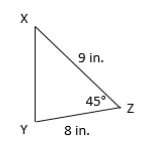We need to find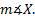,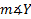, and the length of side z. Let's take them one at a time.

1) First we'll use the Law of Cosines to find the length of side z.

We know we need to use the Law of Cosines because we are have SAS (two sides and the angle in between.)

C will be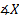or 45°. a and b are 9 and 8. Let's substitute and solve: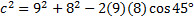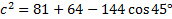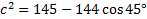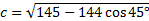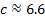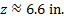2) Now we need to findLet's input our new info into our triangle: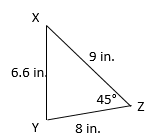We can use either the Law of Sines or the Law of Cosines. Let's use the Law of Sines (rewritten to portray angles X, Y, and Z instead of A, B, and C)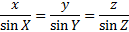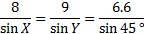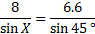8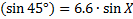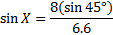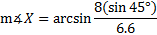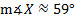3) Find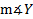Since we know that the three angles of a triangle add up to 180°, we can subtract the two known angles to find the third:

180° - 45° - 59° = 76°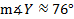Now this answer assumes we found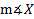correctly. To double check your answer, you can findusing either the Law of Sines or the Law of Cosines. If that answer also comes out around 76°, you can be fairly confident that you did everything correctly. Note, however, that both answers are dependent upon your calculation of side z, and z was rounded to the tenths place. Thus, there will be some variety in the answer due to rounding error. To reduce this, keep the value for z in the memory of your calculator and use this value instead of the rounded 6.6 in the final calculation.

Practice: Solve these triangles. Round all answers to the nearest tenth.

#1-3: Solve triangle XYZ

#4-6: Solve triangle DEF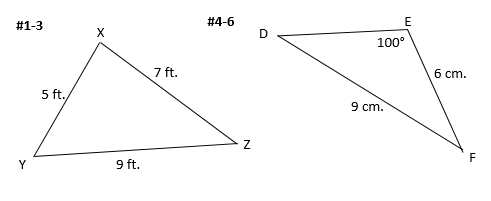Answers: (Note: Answers may vary slightly due to differences in rounding.)

#1-3)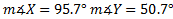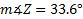#4-6)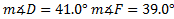f = 5.8 cm.

 Related Links: Math Fractions Factors